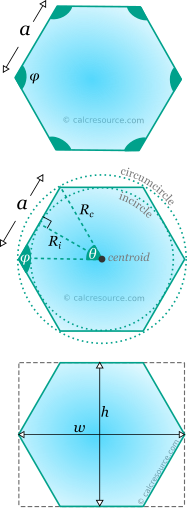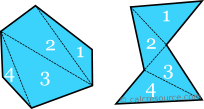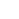## Regular hexagon calculator

This tool calculates the basic geometric properties of a regular hexagon. Regular polygons are equilateral (all sides equal) and their angles are equal too. The tool can calculate the properties of the hexagon, given either the length of its sides or the inradius or the circumradius or the area or the height or the width. Enter below the shape dimensions. The calculated results will have the same units as your input. Please use consistent units for any input.

 Geometric properties: Area = Perimeter = α = Ri = Rc = ADVERTISEMENT Bounding box: Height h = Width w = Angles : deg rad Interior φ = Central θ =## Theoretical background

### Definitions

Hexagon is a polygon with six sides and six vertices. Like any polygon, a hexagon can be either convex or concave, as illustrated in the next figure. When convex, a hexagon (or a polygon in general) has none of its interior angles greater than 180°. To the contrary, a concave hexagon (or polygon) has one or more of its interior angles greater than 180°. A hexagon is called regular when all of its sides and interior angles are equal. Unlike the triangle, having the sides equal does not imply that the interior angles are also equal, since the hexagon may be concave. As demonstrated in the figure below, many possible concave hexagons can be defined, with equal sides, but unequal interior angles (these are called equilateral). Any hexagon that is not regular is called irregular.

The sum of the internal angles of any hexagon, either convex or concave is always 720°. This can be easily be concluded by counting the number of triangles fitting inside the hexagon, by connecting its vertices (avoiding intersections). Indeed, there are 4 triangles. Since he sum of internal angles in one triangle is 180°, 4 triangles, side by side, should measure up to 4x180=720°.The diagonals of a hexagon separate its interior into 4 triangles

### Properties of regular hexagons

#### Symmetry

The regular hexagon features six axes of symmetry. Half of them are passing through diagonally opposite vertices and the remaining through the middles of opposite edges.

#### Interior angle and central angle

Regular polygons have equal interior angles by definition. Since there are six of them in total, it can be concluded that each interior angle of a regular hexagon is equal to:

Drawing straight lines from the center of the hexagon towards each one of its vertices we end up with six identical triangles. The central angle of each triangle is equal to:

The remaining two angles in the triangle are also equal to 60°. These are actually because the diagonals of the hexagon (lines connecting opposite vertices) are also axes of symmetry, thus bisecting interior angle (which is 120°). Therefore, all the six triangles sharing the center of the hexagon as one of their vertices, are identical equilateral triangles.

The interior and central angles are also supplementary, since their sum is 180°:The regular hexagon is composed by six identical equilateral triangles having a common vertex, the polygon centre.

#### Circumcircle and incircle

As happens with any regular polygon, a circle that passes through all six vertices of the hexagon can be drawn. This is the cirmuscribed circle or circumcircle of the polygon. The center of this circle is the center of the hexagon. Likewise, the diagonals of the hexagon are diameters of the circumcircle. The radius of circumcircle is usually called circumradius.

Another circle can also be drawn, that passes through the middles of the hexagon sides. This circle is called inscribed circle or incircle. It is tangent to all six sides and its center coincides with the center of the circumcircle. The radius of incircle is usually called inradius.

The radius of circumcircle and incircle are related to the side length and also among each other. These relationships can be discovered using the properties of the right triangle, whose sides are: the circumradius, the inradius and half the hexagon side, as highlighted in the figure below. Using basic trigonometry we find:

where the central angle and the side length. It turns out that these expressions are valid for any regular polygon, not just the hexagon. We can obtain specific expression for the regular hexagon by setting θ = 60°. These expressions are:

.The circumradius of a regular hexagon is equal to its side.

#### Area and perimeter

The area of a regular hexagon can be found, considering that it is composed by 6 identical equilateral triangles, having sides and height the hexagon inradius (see figure below). The area of each triangle is . Therefore, the total area of the six triangles is:

An approximation of the last equation is:

The perimeter of any N-sided regular polygon is simply the sum of the lengths of all sides: . Therefore, for the regular hexagon :

### Bounding box

The bounding box of a planar shape is the smallest rectangle that encloses the shape completely. The dimensions of this rectangle are defined by the height and t the width of the regular hexagon.

##### Height

The height of the regular hexagon is the distance between two opposite edges. This is equal to twice the inradius :

It is possible to express the height in terms of the circumradius , or equivalently the side length , using the respective analytical expressions for these quantities. The following formulas are derived:

which is approximated to:

##### Width

The width is the distance between two opposite vertices of the regular hexagon (the length of its diagonal). This is equal to twice the circumradius :

Because, , we could write also:

### How to draw a regular hexagon

You can draw a regular hexagon of a given side length , using only a ruler and a compass. Follow the steps described below:

1. First just draw a linear segment whose length is the desired hexagon side length .
2. Then construct a circle, having its center at one end of the linear segment and radius equal to the segment length.
3. Without changing the radius, construct a second circle, having its center at the other end of the linear segment. Two points are defined where this circle intersects with the first one.
4. Using the same radius, place the tip of the compass on either one of the lastly defined points and construct a new circle. A new point is defined at the intersection with the first circle.
5. Repeat the same procedure two more times. Place the tip of the compass on the last point and construct a new circle. A new point is defined at the intersection with the first circle.
6. Six points have been defined so far around the first circle. These are the vertices of the hexagon. Draw linear segments between them and the regular hexagon is now complete. The initial circle is the circumcircle of the hexagon.

The following figure illustrates the drawing procedure step by step.

### Examples

##### Example 1

Determine the circumradius, the inradius and the area of a regular hexagon with side length .

The circumradius and the inradius, in terms of the side length , for the regular hexagon, have been derived in the previous sections. They are:

.

Therefore, we have to simply substitute . Doing so we find the circumradius:

The area of a regular hexagon is given by the equation:

Substituting we find:

##### Example 2 1

How many individual cells may fit in one side of a bee hive frame with dimensions 19''x9''. Assume that each cell is a regular hexagon and that its diagonal is equal to 5.4mm.

The total area of the frame is:

Now we have to find the area of an individual cell. Its diagonal is actually the diameter of the circumcircle, therefore the circumradius is:

However, for a regular hexagon circumradius is also equal to the side length:

For the area of the regular hexagon we use the approximate expression:

Therefore:

A key feature of the regular hexagon is its ability to tile a plane area without leaving any gaps. This property permits us to assume that no space of the hive frame is left unoccupied. In other words, we can find the number of cells in the hive frame, , by dividing the total frame area by the cell area:

By substitution and conversion from square inches to mm2 (i.e.: 1''=25.4mm) we get:

Almost six thousand cells. This number could be a bit lower though, if we take into account that next to the boundaries of the frame, the formation of regular hexagons is impossible. Assuming that one vertical and one horizontal column of cells is wasted, due to this reason, the count would be lower by 140 cells, approximately. Also, take in mind that the cell size can vary. The given size in this example is common for commercially available hive frames. Naturally occurring cells can be smaller.

### Regular hexagon cheat-sheet

In the following table a concise list of the main formulas, related to the regular hexagon is included. Also some approximations that may prove handy for practical problems are listed too.